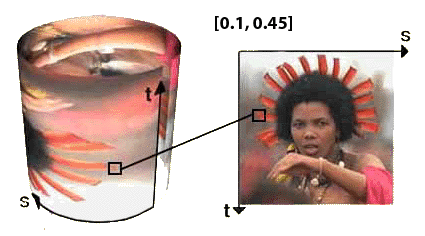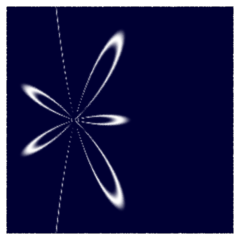### RSLPolar Coordinates & Famous Curves

#### Introduction

This tutorial looks at the basics of creating patterns defined within a 2D polar coordinate system. Using polar coordinates is somewhat unusual because the majority of effects applied by shaders generally depend on the direct use of 2D 'st' texture coordinates and/or the 3D coordinates of points, vectors and normals such as the global variables `P, I` and `N`. Before looking at the use of a 2D polar coordinate system this tutorial clarifies what are generically referred to as `Cartesian` coodinates.

#### Cartesian Coordinates

Locations within Cartesian coordinate systems are measured relative to the intersection of fixed coordinate axes that are perpendicular to each other. For example, figure 1 shows the coordinates (`0.1, 0.45`) of a point within a 2D Cartesian coordinate ('st' texture) system. Although 'st' texture space uses two perpendicular axes it does not mean they must be planar. 2D coordinate systems can be "drapped" around or over a curved surface.Figure 1

Figure 2 shows the coordinates (`1.0, 2.0, 0.7`) of a point within a 3D Cartesian coordinate system.Figure 2

#### 2D Polar Coordinates

Figure 3 illustrates how the location of a point, within a polar coordinate system, is specified by distance (`R`) and angle (`theta`). In the shading language angles are measured in radians not degrees.Figure 3

Relative to the center of the 1 x 1 polygon, shown in figure 3, the coordinates of the hilited box are,
`R` = `0.4`
`theta` = `0.698` (ie. 40 degrees)
Since neither `R` or `theta` are global variables their values must be calculated by converting the 'st' (Cartesian) texture coordinates to their polar equivalents - listing 1.

Listing 1 - polar.h

 ```void cartToPolar2D(float x, y, center, ycenter output varying float R, theta) { float xdiff = x - xcenter, ydiff = y - ycenter; R = sqrt(xdiff * xdiff + ydiff * ydiff); theta = atan(ydiff, xdiff); // -PI to PI radians theta = theta + 2 * PI; // 0 to 2PI radians }```

#### Drawing Curves

The School of Mathematics and Statistics at the University of St.Andrews, Scotland maintains a web site of "Famous Curves". Many of the curves are defined by formula that require the use of polar coordinates. For example, figure 4 uses the polar description of a curve called the "Trisectrix of Maclaurin"Figure 4 - output of the Maclaurin shader

Listing 2 provides the code for the shader that produced the pattern shown above. A sample rib file, listing 3, demonstrates the use of the shader.

Listing 2 - maclaurin.sl

 ```surface maclaurin(float Kfb = 1, /* Fake brightness */ sc = 10, /* [0.01 20] Scaling factor */ a = 2, /* [0 10] Scaling factor */ b = 6, /* [0 10] Lobes coefficient */ stroke = 0.03, /* [0 1] Lobes coefficient */ blur = 0.5, /* [0 1] Softens the stroke */ scenter = 0.5, /* [-2 2] Center in 's' */ tcenter = 0.5; /* [-2 2] Center in 't' */ color bakcolor = color(0,0,0.2), patcolor = 1 /* Color of the stroke */ ) { float R, theta; float ss = s * sc; float tt = t * sc; // Convert the s,t location of the shading point // to polar coordinates. float xdiff = ss - scenter * sc, ydiff = tt - tcenter * sc; R = sqrt(xdiff * xdiff + ydiff * ydiff); theta = atan(ydiff, xdiff); // -PI to PI radians if(theta < 0.0) theta += 2 * PI; // 0 to 2PI radians // Use the Maclaurin formula float r = a * cos(b * theta)/cos(theta); float blend = smoothstep(abs(R - r) - blur, abs(R - r), stroke); Oi = Os; Ci = Oi * mix(bakcolor, patcolor, blend) * Kfb; }```

Listing 3 - maclaurin.rib

 ```Option "searchpath" "shader" "@:PATH_TO_USERS_SHADERS_DIR" Display "maclaurin" "it" "rgb" Format 240 240 1 Projection "perspective" "fov" 20 ShadingRate 1 Translate 0 0 3 Rotate -90 1 0 0 Rotate 0 0 1 0 Scale 1 1 -1 WorldBegin Surface "maclaurin" "float sc" 10 "float a" 2 "float b" 6 Polygon "P" [-0.5 0 -0.5 -0.5 0 0.5 0.5 0 0.5 0.5 0 -0.5] "st" [0 0 0 1 1 1 1 0] WorldEnd```